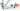# APR to EAR Calculator

Calculate the Effective Annual Rate (EAR) using the Annual Percentage Rate (APR). You can choose the compounding period to be either monthly, quarterly, or semiannually.Equitysim - explore your financial scenarios and make better financial decisions

$\text{EAR} = \left( \left( \frac{\text{APR}}{100 \, n } + 1 \right)^n - 1 \right) \times 100$